# Resources tagged with: Ratio and proportion

Filter by: Content type:
Age range:
Challenge level:

##### Other tags that relate to CSI: Chemical Scene Investigation
STEM - General. Engineering. Maths Supporting SET. Biology. Chemistry. Logo. Ratio and proportion. Exponential and Logarithmic Functions. Physics. Investigations.

### There are 66 results

Broad Topics > Fractions, Decimals, Percentages, Ratio and Proportion > Ratio and proportion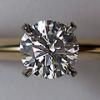### Diamonds Aren't Forever

##### Age 16 to 18 Challenge Level:

Ever wondered what it would be like to vaporise a diamond? Find out inside...### Extreme Dissociation

##### Age 16 to 18 Challenge Level:

In this question we push the pH formula to its theoretical limits.### Striking Gold

##### Age 16 to 18 Challenge Level:

Investigate some of the issues raised by Geiger and Marsden's famous scattering experiment in which they fired alpha particles at a sheet of gold.### Eudiometry

##### Age 16 to 18 Challenge Level:

When a mixture of gases burn, will the volume change?### Gassy Information

##### Age 16 to 18 Challenge Level:

Do each of these scenarios allow you fully to deduce the required facts about the reactants?### Reductant Ratios

##### Age 16 to 18 Challenge Level:

What does the empirical formula of this mixture of iron oxides tell you about its consituents?### Why Multiply When You're about to Divide?

##### Age 16 to 18 Challenge Level:

A brief introduction to PCR and restriction mapping, with relevant calculations...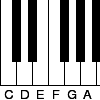### Tuning and Ratio

##### Age 16 to 18 Challenge Level:

Why is the modern piano tuned using an equal tempered scale and what has this got to do with logarithms?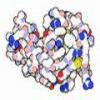### Mixed up Mixture

##### Age 14 to 16 Challenge Level:

Can you fill in the mixed up numbers in this dilution calculation?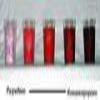### Exact Dilutions

##### Age 14 to 16 Challenge Level:

Which exact dilution ratios can you make using only 2 dilutions?### Investigating the Dilution Series

##### Age 14 to 16 Challenge Level:

Which dilutions can you make using only 10ml pipettes?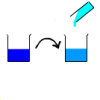### Dilution Series Calculator

##### Age 14 to 16 Challenge Level:

Which dilutions can you make using 10ml pipettes and 100ml measuring cylinders?### Eight Ratios

##### Age 14 to 16 Challenge Level:

Two perpendicular lines lie across each other and the end points are joined to form a quadrilateral. Eight ratios are defined, three are given but five need to be found.### Ratios and Dilutions

##### Age 14 to 16 Challenge Level:

Scientists often require solutions which are diluted to a particular concentration. In this problem, you can explore the mathematics of simple dilutions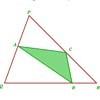### Halving the Triangle

##### Age 16 to 18 Challenge Level:

Draw any triangle PQR. Find points A, B and C, one on each side of the triangle, such that the area of triangle ABC is a given fraction of the area of triangle PQR.### Five Circuits, Seven Spins

##### Age 16 to 18 Challenge Level:

A circular plate rolls inside a rectangular tray making five circuits and rotating about its centre seven times. Find the dimensions of the tray.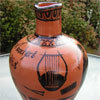### Six Notes All Nice Ratios

##### Age 14 to 16 Challenge Level:

The Pythagoreans noticed that nice simple ratios of string length made nice sounds together.### Another Triangle in a Triangle

##### Age 16 to 18 Challenge Level:

Can you work out the fraction of the original triangle that is covered by the green triangle?### Roasting Old Chestnuts 3

##### Age 11 to 16

Mainly for teachers. More mathematics of yesteryear.### Make Your Own Solar System

##### Age 7 to 16 Challenge Level:

Making a scale model of the solar system### The Fastest Cyclist

##### Age 14 to 16 Challenge Level:

Andy is desperate to reach John o'Groats first. Can you devise a winning race plan?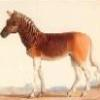### Rarity

##### Age 16 to 18 Challenge Level:

Show that it is rare for a ratio of ratios to be rational.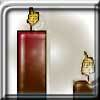### Burning Down

##### Age 14 to 16 Challenge Level:

One night two candles were lit. Can you work out how long each candle was originally?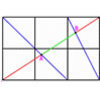##### Age 16 to 18 Challenge Level:

A new problem posed by Lyndon Baker who has devised many NRICH problems over the years.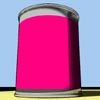### Tin Tight

##### Age 14 to 16 Challenge Level:

What's the most efficient proportion for a 1 litre tin of paint?### Golden Ratio

##### Age 16 to 18 Challenge Level:

Solve an equation involving the Golden Ratio phi where the unknown occurs as a power of phi.### Chord

##### Age 16 to 18 Challenge Level:

Equal touching circles have centres on a line. From a point of this line on a circle, a tangent is drawn to the farthest circle. Find the lengths of chords where the line cuts the other circles.### Napoleon's Hat

##### Age 16 to 18 Challenge Level:

Three equilateral triangles ABC, AYX and XZB are drawn with the point X a moveable point on AB. The points P, Q and R are the centres of the three triangles. What can you say about triangle PQR?### Nutrition and Cycling

##### Age 14 to 16 Challenge Level:

Andy wants to cycle from Land's End to John o'Groats. Will he be able to eat enough to keep him going?### Bus Stop

##### Age 14 to 16 Challenge Level:

Two buses leave at the same time from two towns Shipton and Veston on the same long road, travelling towards each other. At each mile along the road are milestones. The buses' speeds are constant. . . .### Plane to See

##### Age 16 to 18 Challenge Level:

P is the midpoint of an edge of a cube and Q divides another edge in the ratio 1 to 4. Find the ratio of the volumes of the two pieces of the cube cut by a plane through PQ and a vertex.### A Scale for the Solar System

##### Age 14 to 16 Challenge Level:

The Earth is further from the Sun than Venus, but how much further? Twice as far? Ten times?### Areas and Ratios

##### Age 16 to 18 Challenge Level:

Do you have enough information to work out the area of the shaded quadrilateral?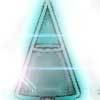### Conical Bottle

##### Age 14 to 16 Challenge Level:

A right circular cone is filled with liquid to a depth of half its vertical height. The cone is inverted. How high up the vertical height of the cone will the liquid rise?### Slippage

##### Age 14 to 16 Challenge Level:

A ladder 3m long rests against a wall with one end a short distance from its base. Between the wall and the base of a ladder is a garden storage box 1m tall and 1m high. What is the maximum distance. . . .### Speeding Boats

##### Age 14 to 16 Challenge Level:

Two boats travel up and down a lake. Can you picture where they will cross if you know how fast each boat is travelling?### Mixing More Paints

##### Age 14 to 16 Challenge Level:

Can you find an efficent way to mix paints in any ratio?### Points in Pairs

##### Age 14 to 16 Challenge Level:

Move the point P to see how P' moves. Then use your insights to calculate a missing length.### Triangle in a Triangle

##### Age 14 to 16 Challenge Level:

Can you work out the fraction of the original triangle that is covered by the inner triangle?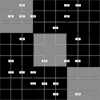### Ratio Sudoku 2

##### Age 11 to 16 Challenge Level:

A Sudoku with clues as ratios.### Orbiting Billiard Balls

##### Age 14 to 16 Challenge Level:

What angle is needed for a ball to do a circuit of the billiard table and then pass through its original position?### At a Glance

##### Age 14 to 16 Challenge Level:

The area of a regular pentagon looks about twice as a big as the pentangle star drawn within it. Is it?### Circuit Training

##### Age 14 to 16 Challenge Level:

Mike and Monisha meet at the race track, which is 400m round. Just to make a point, Mike runs anticlockwise whilst Monisha runs clockwise. Where will they meet on their way around and will they ever. . . .### Rhombus in Rectangle

##### Age 14 to 16 Challenge Level:

Take any rectangle ABCD such that AB > BC. The point P is on AB and Q is on CD. Show that there is exactly one position of P and Q such that APCQ is a rhombus.### Golden Thoughts

##### Age 14 to 16 Challenge Level:

Rectangle PQRS has X and Y on the edges. Triangles PQY, YRX and XSP have equal areas. Prove X and Y divide the sides of PQRS in the golden ratio.##### Age 14 to 16 Challenge Level:

Four jewellers share their stock. Can you work out the relative values of their gems?### Trapezium Four

##### Age 14 to 16 Challenge Level:

The diagonals of a trapezium divide it into four parts. Can you create a trapezium where three of those parts are equal in area?### From All Corners

##### Age 14 to 16 Challenge Level:

Straight lines are drawn from each corner of a square to the mid points of the opposite sides. Express the area of the octagon that is formed at the centre as a fraction of the area of the square.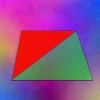### Same Height

##### Age 14 to 16 Challenge Level:

A trapezium is divided into four triangles by its diagonals. Can you work out the area of the trapezium?### One and Three

##### Age 14 to 16 Challenge Level:

Two motorboats travelling up and down a lake at constant speeds leave opposite ends A and B at the same instant, passing each other, for the first time 600 metres from A, and on their return, 400. . . .